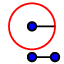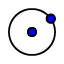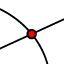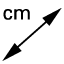# Perpendicular Bisectors and Triangle Circumcenters

A circumcenter is located at the point of intersection of the perpendicular bisectors of all three sides of a triangle. Follow the instructions to construct the perpendicular bisector of segment AB and then answer the questions that follow. *A note before we begin. Geogebra does contain many shortcut commands to make constructions easier (such as finding the midpoint or angle bisector with a single command). For the purpose of these exercises though, we are going to try to make them more similar to a compass and straightedge construction done by hand by including the construction of circles (arcs).*
To find the perpendicular bisector below, we are going to construct two congruent circles (this will be easier in Geogebra that constructing arcs which are nothing more than a portion of a circle). Each circle will be centered on opposite endpoints of segment AB. We are going to start by selecting the Compass tool. The tools are in stacked menus. To find the compass tool, first click on the Circle with Center through Pointand then click on Compass. With this tool, you will select two points to use as the width of your compass, and then select a third point to be the center of the circle. So, after your have selected Compass, click on point A and then point C (I placed point C on the segment more than half the distance from A to B to help you create consistent circles. Then click on point A again in order to center the circle there. Repeat this process to place a second circle of equal size centered on point B. Notice that the circles intersect at two points. We can place points at these intersections. To do this, we will use the Intersect tool. This is found under the Point tool. Select Intersectand then select the two circles. We can now use these points to make the perpendicular bisector. To do this, select the Line tool. Then click on each of the points you created at the intersections of the circles. You now have the perpendicular bisector.

## Perpendicular Bisector

Which of the following points does the perpendicular bisector go through?

Select all that apply
• A
• B
• C
• D

## Points on Perpendicular Bisectors

In the applet below, point C lies on the perpendicular bisector of segment AB. Move point C up and down the perpendicular bisector. Notice the lengths of AC and BC as you do. Answer the question that follows.

## Points on a Perpendicular Bisector

Based on your observation as you moved point C above, what can we say regarding ANY point on a perpendicular bisector as it relates to the end points of the segment?

## Circumcenters

To find the circumcenter of a triangle, we need to find the intersection of the three perpendicular bisectors of the sides of the triangle. In the exercise below. Find the circumcenter using the method described above. To makes things a little neater and easier, note that you only need to find the intersection of two perpendicular bisectors in order to find the circumcenter. Place a point at the intersection where the circumcenter is located using the Intersect toolFind the distance from the circumcenter to each of the vertices of the triangle. This can be done using the Distance or Length tooland clicking on the circumcenter and then the vertex. This is found in the menu under the Angle tool.

What are the coordinates of the circumcenter?

What is the distance from the circumcenter to vertex A?

What is the distance from the circumcenter to vertex B?

What is the distance from the circumcenter to vertex C?

Go back to the exercise above and construct a circumscribed circle around the triangle. Do this using the Circle through a point tool. Click on the circumcenter as your center of the circle, and then click on vertex A of the triangle. What other points does the circle go through?